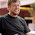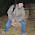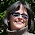## Tuesday, August 24, 2010

### Why Python's Integer Division Floors

I was asked (again) today to explain why integer division in Python returns the floor of the result instead of truncating towards zero like C.

For positive numbers, there's no surprise:

>>> 5//2
2

But if one of the operands is negative, the result is floored, i.e., rounded away from zero (towards negative infinity):

>>> -5//2
-3
>>> 5//-2
-3

This disturbs some people, but there is a good mathematical reason. The integer division operation (//) and its sibling, the modulo operation (%), go together and satisfy a nice mathematical relationship (all variables are integers):

a/b = q with remainder r

such that

b*q + r = a and 0 <= r < b

(assuming a and b are >= 0).

If you want the relationship to extend for negative a (keeping b positive), you have two choices: if you truncate q towards zero, r will become negative, so that the invariant changes to 0 <= abs(r) < otherwise, you can floor q towards negative infinity, and the invariant remains 0 <= r < b. [update: fixed this para]

In mathematical number theory, mathematicians always prefer the latter choice (see e.g. Wikipedia). For Python, I made the same choice because there are some interesting applications of the modulo operation where the sign of a is uninteresting. Consider taking a POSIX timestamp (seconds since the start of 1970) and turning it into the time of day. Since there are 24*3600 = 86400 seconds in a day, this calculation is simply t % 86400. But if we were to express times before 1970 using negative numbers, the "truncate towards zero" rule would give a meaningless result! Using the floor rule it all works out fine.

Other applications I've thought of are computations of pixel positions in computer graphics. I'm sure there are more.

For negative b, by the way, everything just flips, and the invariant becomes:

0 >= r > b.

So why doesn't C do it this way? Probably the hardware didn't do this at the time C was designed. And the hardware probably didn't do it this way because in the oldest hardware, negative numbers were represented as "sign + magnitude" rather than the two's complement representation used these days (at least for integers). My first computer was a Control Data mainframe and it used one's complement for integers as well as floats. A pattern of 60 ones meant negative zero!

Tim Peters, who knows where all Python's floating point skeletons are buried, has expressed some worry about my desire to extend these rules to floating point modulo. He's probably right; the truncate-towards-negative-infinity rule can cause precision loss for x%1.0 when x is a very small negative number. But that's not enough for me to break integer modulo, and // is tightly coupled to that.

PS. Note that I am using // instead of / -- this is Python 3 syntax, and also allowed in Python 2 to emphasize that you know you are invoking integer division. The / operator in Python 2 is ambiguous, since it returns a different result for two integer operands than for an int and a float or two floats. But that's a totally separate story; see PEP 238.

#### 18 comments:

1.Tim is right to be worried about extending the truncate-toward-negative-infinity rule to floating-point numbers. The reason is that if a%b always has the sign of a when a's and b's signs differ, then a%b can always be represented exactly as a floating-point number if a and b can be represented exactly--at least, in every floating-point representation system I've seen. If, however, a%b takes on the sign of b when the signs differ, there are values of a and b that can preclude a%b from being represented exactly.

2.Should note that C Classic didn't define the sign of the result, but did require that

a == (a/b)*b + a%b

hold whenever a/b was representable. Not one C programmer in a million knew that, though ;-) C99 finally did insist on giving the wrong answer, seemingly just to be compatible with FORTRAN.

I've never seen an integer use case where the wrong answer was helpful. Use cases where the right answer are helpful abound. For example, when trading equity options the 3rd Friday of the month is a crucial date, and

import datetime
FRIDAY = 4
# Return date of third Friday of month.
def fri3(year, month):
d = datetime.date(year, month, 1)
diff = (FRIDAY - d.weekday()) % 7 + 14
return d + datetime.timedelta(days=diff)

is screamingly natural. Weekdays, hours on a 24-clock, minutes, seconds, months in a year, year numbers in a century ... many things related to time are naturally represented by the ordinals in range(N) for some N, repeated endlessly. Using the right definition of % allows uniform code to move forward or backward in such sequences.

But for floats it's usually most useful to have

0 <= a%b <= abs(b/2)

and damn the signs. The mathematical result is exactly representable (ark's point) under that constraint too, and it caters to that floating mod is most often used for range reduction (so that it's helpful for the result to have the smallest absolute value possible).

The integers are a subset of the reals in math, but that has nothing to do with floats ;-)

3.LOL - it's wonderfully ironic that the commenting system destroyed the whitespace in my Python code snippet :-)

4.Thanks a lot for taking the time to answer this :)
Now everyone on the IRC channel owns me a cookie !

And the explanation is very interesting, never thought about it this way before.

5.Thanks Twitterers @dgou and @schuetzdj for reporting a text mistake; I've fixed it now!

6.Thanks a lot for this answer =]
Finally I'll be able to stop wondering why Python integer division was implemented that way.
But now, I owe WapiFlapi a cookie...

7.The CDC behavior of 60 1's being negative zero indicates one's complement representation, not sign magnitude. Ah, the days of the Cyber-70!

8.Not to argue that this was a bad decision, but I just wanted to report that this bit me when trying to convert integral cents into dollars and cents. cents%100, cents/100 is a construct I learned in my very early days of programming. It didn't occur to me that this wouldn't work in python, but it blew up rather spectacularly with negative amounts.

Now, the Python standard library also has the very excellent Decimal class, which is what we use internally to represent all money amounts. So the fix was to simply construct the Decimal, than divide it by 100, which is a shorter and cleaner code. So, the story has a very happy ending =)

9.It might be worth noting, that divmod for Decimal behaves different than divmod for int / float when used with negative numbers.

10.For the benefit of those wondering which approach to take for their own programming language, consider that there are cases where the floor integer division doesn't obey the normal rules of algebra as well as truncating integer division; some apparently equivalent expressions will give different results:

-5//2 * -1 == -2, but -1 * -5 // 2 == -3.

-5//2 + 5//2 == -1, but (-5 + 5)//2 == 0.

So, don't be afraid to adopt the same approach as C, Javascript, and so on worrying that it's less correct or more error prone. Probably neither approach is really "better", it's just a matter of preference.

See http://en.wikipedia.org/wiki/Modulo_operation for a list of programming languages and how they chose to do integer division.

11.@Dobes: indeed. Also note that Python's approach is not ideal when generalized to floats. Tim Peters was the first to point this out to me.

12.Just crap and a very bad decision. Just like 0.1 + 0.2 is not 0.3 in python. Float representation is -horrible- in python. May be "mathematically" correct, it doesn't make sense to 99% of people and just bugs 99% of people, no matter what you explain here

13.Float representation is horrible everywhere. And .1+.2 is not .3 almost everywhere.

14.@Dobes: "The normal rules of algebra" is an ill-defined phrase when discussing integer division. The integers are a ring not a field.

Associativity breaks for any implementation of integer division. Consider (5 + 1)//2 vs. 5//2 + 1//2

The fact that there is one special case when associativity works the way someone might naively expect in C when in general the "normal rules of algebra" are disobeyed, is not really an advantage of C. There is practically no conceivable situation in which you would want associativity exactly for the case of inverses. However, there is certainly a conceivable case where you'd hope it would break: i.e. if you happen to think of a test case that only tests for associativity with inverses. Whatever code included your mistaken notion of how division should associate in C would have been a lot harder to debug than it is in a language like Python when it "breaks" for the obvious test case just as much as it does for all sorts of real scenarios.

Floats on the other hand... well, the original implementation is so screwed up that it's not salvageable. If there were a few invisible trailing bits that got computed with addition and multiplication, but were ignored with comparisons,or if they had been designed to behave like a field, you could say without any reservation that Python's implementation is wrong and that C's implementation is right. As things stand, they're both wrong, and C/javascript's implementation is probably slightly less wrong for floats.

Python's implementation for ints is the correct one. It respects the rules of mathematics much more thoroughly than C's because, unlike integer division, the modulo operation does have well-defined algebraic properties.

When you are dealing with the field that is the natural numbers modulo 7, for example, is always correct under Pythons implementation; whereas, it is correct only as long as your values are positive for C's, which never happens because C's implementation returns negative integers even if all of the ones you started with were positive. grr, (I've seen numerous non-theoretical use-cases for why you would want this. The most obvious have to do with positioning graphical components or with ABCDEFG testing -- a faster way of doing AB testing.)

If you take a closer look at the list you linked to, you will notice that every language that was designed by people who really care about math has an operator either called "mod" or called "%" that does exactly the same thing as Python's implementation. There is some mixture among the quick and dirtier hacker languages. (All the languages with math in their name, Haskell, OCaml, Common Lisp, SML, R, Clojure, maple goes a step further). Every programming language I've ever heard of a mathematician actually using does it this way. Certainly all of the programming languages that mathematicians have written.

If you are writing your own programming language and you want to decide how to implement integer division, please stick with the way that all of the mathematicians use. There are very good reasons why they have decided that one particular implementation and not the other is correct.

15.Thanks. I'm still not sure I understand 100% (I'm here from Coursera, so I'm still learning).

FYI - "Other applications I've though of ..."

Should be "...thought of..."

:)

16.And here I thought it was because you could sometimes replace division by arithmetic shift right (with two's complement). Silly me.

17.And here I thought it was because you could sometimes replace division by arithmetic shift right (with two's complement). Silly me.

18.Another more general example where this is useful is iterating backwards and wrapping over a list (this can also be done with itertools but that's a bit more verbose and complex):

i = end = 15
while True:
val = mylist[i]
i-=1
if i==end: break

Note: Only a member of this blog may post a comment.